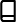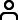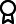Journal metrics
See full report
Acceptance rate25%
Submission to final decision72 days
Acceptance to publication21 days
CiteScore1.900
Journal Citation Indicator0.430
Impact Factor1.2

### On Bernstein’s Problem of Complete Parabolic Hypersurfaces in Warped Products

####Journal profile

Advances in Mathematical Physics publishes papers that seek to understand mathematical basis of physical phenomena, and solve problems in physics via mathematical approaches.

####Editor spotlight

Chief Editor, Prof Di Matteo (Department of Mathematics, King’s College London), engages in world-leading multidisciplinary and data-driven research focussed on the analysis of complex data from the perspective of a statistical physicist.

####Special Issues

Do you think there is an emerging area of research that really needs to be highlighted? Or an existing research area that has been overlooked or would benefit from deeper investigation? Raise the profile of a research area by leading a Special Issue.

## Latest Articles

More articles
Research Article

### Numerical Solutions of Duffing Van der Pol Equations on the Basis of Hybrid Functions

In the present work, a new approximated method for solving the nonlinear Duffing-Van der Pol (D-VdP) oscillator equation is suggested. The approximate solution of this equation is introduced with two separate techniques. First, we convert nonlinear D-VdP equation to a nonlinear Volterra integral equation of the second kind (VIESK) using integration, and then, we approximate it with the hybrid Legendre polynomials and block-pulse function (HLBPFs). The next technique is to convert this equation into a system of ordinary differential equation of the first order (SODE) and solve it according to the proposed approximate method. The main goal of the presented technique is to transform these problems into a nonlinear system of algebraic equations using the operational matrix obtained from the integration, which can be solved by a proper numerical method; thus, the solution procedures are either reduced or simplified accordingly. The benefit of the hybrid functions is that they can be adjusted for different values of and , in addition to being capable of yield greater correct numerical answers than the piecewise constant orthogonal function, for the results of integral equations. Resolved governance equation using the Runge-Kutta fourth order algorithm with the stepping time 0.01 s via numerical solution. The approximate results obtained from the proposed method show that this method is effective. The evaluation has been proven that the proposed technique is in good agreement with the numerical results of other methods.

Research Article

### Mixed Lump-Stripe Soliton Solutions to a New Extended Jimbo-Miwa Equation

In this paper, the localized properties of lump and interaction solutions to a new extended Jimbo-Miwa (EJM) equation are studied. Based on the Hirota bilinear method and the test function method, the exact solutions of the EJM equation are discussed; the lump soliton solution, lump-kink soliton solution, and periodic lump solution are obtained. Furthermore, the dynamic properties of the obtained solutions are also discussed by graphical simulation. As far as we know, the obtained results have not been reported.

Research Article

### A Study on Regular Domination in Vague Graphs with Application

Vague graphs (VGs), which are a family of fuzzy graphs (FGs), are a well-organized and useful tool for capturing and resolving a range of real-world scenarios involving ambiguous data. In graph theory, a dominating set (DS) for a graph is a subset of the vertices such that every vertex not in is adjacent to at least one member of . The concept of DS in FGs has received the attention of many researchers due to its many applications in various fields such as computer science and electronic networks. In this paper, we introduce the notion of -Regular vague dominating set and provide some examples to explain various concepts introduced. Also, some results were discussed. Additionally, the -Regular strong (weak) and independent strong (weak) domination sets for vague domination set (VDS) were presented with some theorems to support the context.

Research Article

### On the Characterization of Antineutrosophic Subgroup

This article gives some essential scopes to study the characterizations of the antineutrosophic subgroup and antineutrosophic normal subgroup. Again, several theories and properties have been mentioned which are essential for analyzing their mathematical framework. Moreover, their homomorphic properties have been discussed.

Research Article

### Effect of Classical and Quantum Superposition of Atomic States on Quantum Correlations

In this paper, we report the effect of classical and quantum superposition of atomic states on quantum correlations. Coupled photon pairs generated in a ladder quantum beat laser using coherent-induced classical field and atomic state coherent superposition are considered. Once the quantum coherence get sufficient time, it can generate quantum correlations that include quantum discord, quantum entanglement, and quantum steering, which quickly increase with time until they get their maximum strength. Their strength can be improved further by increasing the number of superposed atoms per unit time, selecting an appropriate amplitude of the classical fields, and managing the amount of temperatures and phase fluctuations. In particular, two-way quantum steering, which is a guarantee for the existence of quantum discord and quantum entanglement, can be achieved by increasing the rate of atomic injection from 2 kHz to 25 kHz even if the mean temperature of the heat bath is considered. The maximum achievable strength of quantum correlations is enhanced by increasing the rate of atomic injection and choosing an appropriate parameters of the coherent-induced classical field in the open quantum system which is treated by using the density operator approach.

Research Article

### Position Vectors of the Natural Mate and Conjugate of a Space Curve

The concept of the natural mate and the conjugate curves associated to a smooth curve in Euclidian 3-space were introduced initially by Dashmukh and others. In this paper, we give some extra results that add more properties of the natural mate and the conjugate curves associated with a smooth space curve in . The position vectors of the natural mate and the conjugate of a given smooth curve are investigated. Also, using the position vector of the natural mate, the necessary and sufficient condition for a smooth given curve to be a Bertrand curve is introduced. Moreover, a new characterization of a general helix is introduced.Journal metrics
See full report
Acceptance rate25%
Submission to final decision72 days
Acceptance to publication21 days
CiteScore1.900
Journal Citation Indicator0.430
Impact Factor1.2Author guidelinesEditorial boardDatabases and indexingAuthor guidelinesEditorial boardDatabases and indexing

Article of the Year Award: Outstanding research contributions of 2021, as selected by our Chief Editors. Read the winning articles.